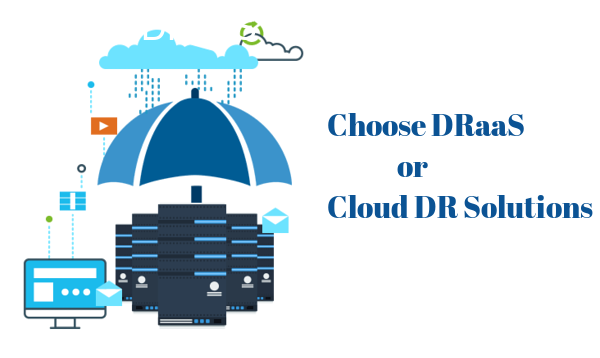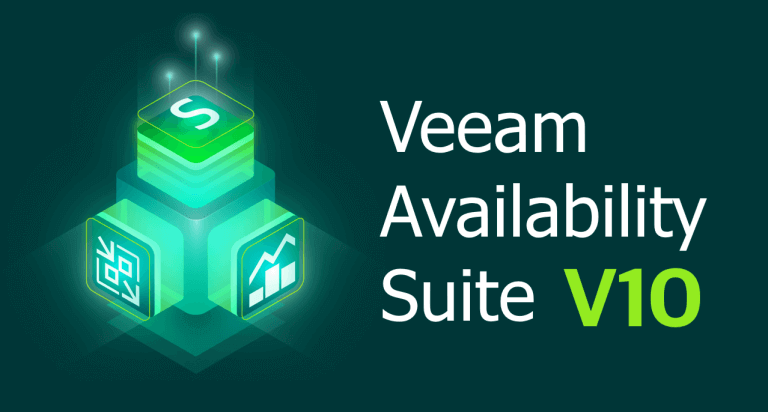# Solutions

We understand the criticality of the move to the cloud and how vital data is to our customers, their customers, and the world. Cloud, multi-cloud, on-premises, virtual and physical servers: our solutions Backup, replicate, and enable cloud mobility. In partnership with Veeam, Truth Technology Inc. offers broad data management and visibility/control capabilities and an extensive orchestration engine to facilitate disaster recovery (DR).### SIMPLE

Granular Recovery, Analytics, Scalable Architecture

x (x)

x (x)

x (x)

x (x)

### RELIABLE

100% tested, portable data format, “it just works”

x (x)

### FLEXIBLE

x (x)## Veeam Availability Suite

Availability for Any App, Any Data, Across Any Cloud

Veeam® Availability Suite™ delivers Availability for ALL workloads — virtual, physical, and cloud — from a single management console. Veeam helps organizations meet today's service-level and data center Availability objectives for the growing enterprise. Veeam Availability Suite is the premier solution providing the superior data protection capabilities of Veeam Backup & Replication™, paired with the advanced monitoring and reporting of Veeam ONE™ for holistic coverage of all workloads.

• Veeam Availability Suite

• Veeam Backup & Replication

• Veeam ONE

x (x)

## Backup Solutions for Small Business

x (x)

x (x)

x (x)

x (x)

x (x)

x (x)

Veeam® Backup Essentials™ delivers powerful, affordable, and easy-to-use backup, recovery, monitoring, and reporting for VMware vSphere, Microsoft Hyper-V, and Nutanix AHV, as well as physical and cloud workloads (including AWS and Azure).

This small business backup solution is designed for companies with less than 250 employees and environments no larger than 6 CPU Sockets or 50 Instances. At the same time, Veeam Backup Essentials delivers the enterprise-level functionality of Veeam Availability Suite™ with savings of up to 60%.

x (x)
x (x)
x (x)
x (x)

## Trusted by 343,000 CustomersWorldwide

x (x)

x (x)

x (x)

x (x)

x (x)

x (x)Royal Caribbean Cruies, Ltd.

x (x)

x (x)

x (x)

x (x)

x (x)

x (x)

x (x)

x (x)

x (x)

x (x)

x (x)

x (x)

x (x)

x (x)

x (x)

x (x)

x (x)

x (x)

x (x)

x (x)

x (x)

x (x)

x (x)

x (x)

x (x)

x (x)

x (x)

x (x)

x (x)

x (x)

x (x)

x (x)

x (x)

x (x)

x (x)

x (x)

x (x)

x (x)

x (x)

x (x)

x (x)

x (x)

x (x)

Royal Caribbean Cruises Ltd. sets sail withbackup and Availability from Veeam

x (x)

x (x)

x (x)

x (x)

x (x)

x (x)

x (x)Telecom Italia

x (x)

x (x)

x (x)

x (x)

x (x)

x (x)

x (x)

x (x)

x (x)

x (x)

x (x)

x (x)

x (x)

x (x)

x (x)

x (x)

x (x)

x (x)

x (x)

x (x)

x (x)

x (x)

x (x)

x (x)

x (x)

x (x)

x (x)

x (x)

x (x)

x (x)

x (x)

x (x)

x (x)

x (x)

x (x)

x (x)

x (x)

x (x)

x (x)

Telecom Italia (TIM) partners with Veeam to deliver high Availability services to their customers

x (x)

x (x)WWF Australia

x (x)

x (x)

x (x)

x (x)

x (x)

x (x)

x (x)

x (x)

x (x)

x (x)

x (x)

x (x)

x (x)

x (x)

x (x)

x (x)

x (x)

x (x)

x (x)

x (x)

x (x)

x (x)

x (x)

x (x)

x (x)

x (x)

x (x)

x (x)

x (x)

x (x)

x (x)

x (x)

x (x)

x (x)

x (x)

x (x)

x (x)

x (x)

x (x)

Veeam Protects WWF-Australia’s data so they can continue to protect our biodiversity and natural environment

x (x)

x (x)

x (x)